CS 559: Computer Graphics
Fall 2001

CS 559 Home
 Calendar Resources Assignments Projects 1 2 3

## A Brief Tutorial On Interpolation for Image Scaling

Michael Gleicher, 10/12/99

When an image is scaled up to a larger size, there is a question of what to do with the new spaces in between the original pixels. Here, we briefly examine some of the differences.

### Resampling in 1D.

To begin, let's consider the 1D case of a signal (call it f) that we would like to dialate (expand in time) by a factor of 2 (call the resulting signal g). This means that

g(t) = f(t/2)

We must consider the problem that these signals are uniformly sampled (at integer values of t). The issue arrises that for some samples that we would like to have of g (namely the odd integers) do not correspond to a sample of f. As we know from our discussions of sampling theory, there is no way to know what the signal does in-between samples.

Theoretically, what we would like to do is construct a continuous representation for f, and then sample that. If we knew that the signal was sampled properly (e.g. the original signal had no frequencies higher than 1/2, which is the Nyquist rate for this sampling period), then we could do an "ideal" reconstruction.

The theoretical process is a good hint at what the "right" answer is: we should create g such that it has no newer high frequencies. Of course, that answer is only right if the original signal was properly sampled. In practice, the "right" answer may be a matter of artistic taste.

To look at a specific example, let's consider a simple f that is a triangle wave with sampled values [0 2 4 6 4 2 0 2 4 6 4 2 0]. This gives us a picture like:What we'd like to do is double the time, which means that we know the even samples.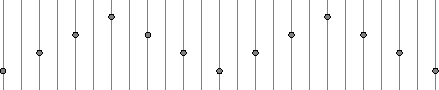So we need to know what happens "in between" these samples. If we had the original signal that f was sampled from, we could sample the signal at all of the desired points.Which of course would have required us to have the original signal (or to at least reconstruct it).

With the numbers, there are two obvious choices:

• Simply double each sample (e.g. repeat it twice). So that our original signal will become [0 0 2 2 4 4 6 6 4 4 2 2 0 0 2 2 4 4 6 6 4 4 2 2 0 0].
• Make the new samples half way between the previous samples [0 1 2 3 4 5 6 5 4 3 2 1 0 1 2 3 4 5 6 5 4 3 2 1 0]. As it turns out, this is exactly the right answer for the picture drawn above. However, this is a fortunate coincident.

The first version we call value replication (or pixel replication) in the case of images. The second is interpolation.

Pixel replication is sometimes called "nearest neighbor" because it picks the sample closest to the value we're looking for. In the case of doubling the image size, we chose to pick the lower value in the case of a tie (e.g. when we look for a value for time = 1.5, we pick sample 1). For an example where this makes a difference consider tripling the time. In this case, we would want to look for time values of 1 1/3 and 1 2/3, which are closest to 1 and 2 respectively.

The halfway method is interpolation. More generally, we blend the nearby samples. The simple way to do this is to draw a line between the two samples and pick the value along the line. This is a simple form of reconstruction. In equation form, we might say

f(t) = s * f(floor(t)) + (1-s) * f(ceil(t))

where floor is the function that picks the largest integer smaller than t, ceil is the "ceiling" function that picks the smallest integer larger than t, and s is t-floor(t), or the distance between t and the sample. This is linear interpolation since we are fitting straight lines between the samples.

Now you might wonder which of the two methods described so far is "right". The answer is "it depends." Suppose we have the triangle wave, and we triple the time (rather than just doubling it). We get two very different looking results: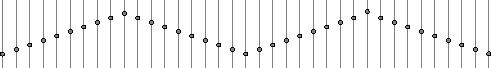for linear interpolation, and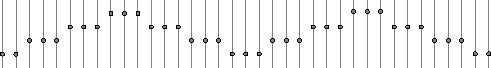for nearest neighbor. Since in this example, we knew that we wanted a triangle wave, one is clearly better than the other. However, if we just had the sampled signal, it might really have been the "jaggy" stairstep. For example, suppose we have a square wave [0 0 1 1 0 0 1 1 0 0 1 1]. In this case, using linear gives an overly smooth result, while nearest neighbor gives a square wave. Nearest neighbor intepolation gives us a square wave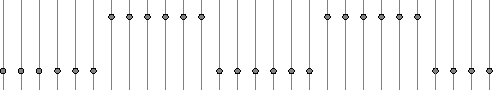while linear interpolation gives us something that might be too smooth.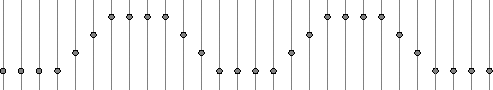Bi-Cubic interpolation achieves results between these two choices. It estimates how sharp and edge there should be by estimating the derivatives at each sample and then fitting a cubic curve between the samples.

### Reconstruction Kernels in 1D

Before moving on to 2D, let's consider a slightly non-intuitive way to implement these methods.

If we take our original samples and "space them out," we get a chain of spikes. Going back to our original example triangle wave [0 2 4 6 4 2 0 2 4 6 2 0], we would just put in zeros in the length doubled version, e.g. [0 0 2 0 4 0 6 0 4 0 2 0 0 0 2 0 4 0 6 0 4 0 2 0]. If we filter this "spike chain" we then get the reconstruction processes described above. By choosing the correct filters, we can different types of reconstruction. For example, nearest neighbor interpolation for size doubling can be implemented by the reconstruction kernel [0 1 1]. The linear interpolation can be implemented by the kernel [.5 1 .5].

For other spacings, we just use other kernels. For example, the nearest neighbor kernel for tripling is [1 1], and the linear interpolation kernel is 1/3 [1 2 3 2 1]. Other kernels give different reconstructions. For example, we might use the kernel 1/6 [1 5 6 5 1].

This implementation has several advantages. One, it gives a uniform way to implement lots of different interpolation types. By choosing the reconstruction kernels, we can get different types of results. Even the bicubic interpolation described above can be implemented this way. Second, it corresponds more closely with the theory of signal reconstruction, which makes design of the reconstruction kernels possible. Third, with a uniform method for kernel design, it is easier to extend this method to different scaling sizes and to 2D.

### Scaling in 2D

Everything we said in 1D also applies in 2D. The biggest difference is that pixels have more neighbors. For example, consider enlarging this image by a factor of 2. I have given some of the pixels 1 character names for future reference.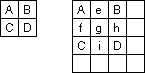Notice that pixel e has neighbors A and B, while pixel g has 4 neighbors. For the pixels with 2 neighbors, the methods we discussed in 1D apply. For those with 4 neighbors, we need to do something different.

The nearest neighbor process has an obvious extension. Linear interpolation requires an extension into two dimensions. We linearly interpolate along each dimension, so the process is called bi-linear intepolation. For the doubling case above, the pixel e would be halfway between A and B (by linear interpolation). Similarly pixels f, h, and i can be found. Pixel g is then halfway between e and i (or equivalently, between f and h). Since e = 1/2 (A+B), and i=1/2(C+D), g=1/4(A+B+C+D).

These reconstructions can be done using the same spike generation and reconstuction filter kernels as done in 1D, except that the kernels are now 2D. In the above example, the "spike" image would be: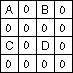The reconstruction kernels for pixel doubling is

 1 1 1 1

for nearest neighbor, and

 1/4 1/2 1/4 1/2 1 1/2 1/4 1/2 1/4

for linear interpolation.

Note: while the linear interpolation kernel may resemble the binomial kernels, they are not the same as the sizes grow. The binomial kernels are more rounded.

### Trying this out

You can try this out using Photoshop or PaintShop Pro. Pick an image,and then use the resize operator to make it bigger. Each program lets you choose between nearest neighbor, bilinear, and bicubic interpolation.

Showing the results here is pretty pointless since the web browsers and web image formats can lose the details of images.## CS559 Web

Home Resources Assignments Tools
Calendar Policies Projects 1 2 3   Examples
Copyright (C) 2001 by Michael Gleicher